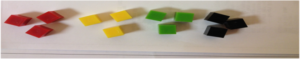What is multiplication?

Multiplication is when we know the number of groups and the size of the equal groups, but the total amount is unknown. Students are expected to use the actions in a story problem to directly model what is happening and find the product.What strategies will lead to students understanding multiplication?

Direct modeling with manipulatives- When students are starting to understand multiplication, they will solve story problems with manipulatives. Have various manipulatives available for students to use. When students directly model with manipulatives, they will show exactly what is happening in the problem to solve. As students are directly modeling, we want to pose questions to get them to think deeper about what’s happening and how they got their answer.
o What do your groups represent?
o What do the cubes in each group represent?
o How does your model relate to the story problem?

Example of direct modeling of a multiplication story problem:Equal groups with quick pictures- With this strategy, students are still directly modeling what is happening in the problem, but they are now representing that model with a picture.Number Line- Students can make connections with their manipulatives and pictures to help them see how number lines can help them solve multiplication problems. The number of groups is represented by the number of jumps and the size of the groups is represented by the size of the jumps. The number at the end of the equal jumps represents the product.Repeated Addition- Students will begin making the connection that repeated addition will help them solve. When students use repeated addition they use the number that is in each group to add repeatedly. The number of groups will tell them how many times to add the number.

3 + 3 + 3 + 3

Skip Counting- As students understanding of multiplication progress, another strategy they will discover is skip counting. They will skip count the number that is in each equal group, and know when to stop based on the number of groups.

3, 6, 9, 12

Multiplication Sentence- All of the strategies discussed above will help students understand the meaning of a multiplication sentence. Once understanding of multiplication begins to build, students should be expected to write multiplication sentences that match their strategy.

4 x 3 = 12

Anchor chart that could be used as students discover the multiplication strategies:What can I do as the parent to support my child’s understanding of multiplication?

Encourage use of models. Make sure not to rush your child to solve without models. With repeated practice and exposure to multiplication, children will naturally become more efficient.

Ask questions that encourage your child to understand why they created the model they did or helps them think deeper about why they multiplied:
o How does your model relate to the problem?
o Equal groups models: What do your groups represent? What do the dots in each group represent?
o Number line model: What does each jump represent? How did you know the value of each jump? What does the number of jumps represent?

For more example division problems visit this website: https://www.ixl.com/math/grade-3/multiplication-word-problems